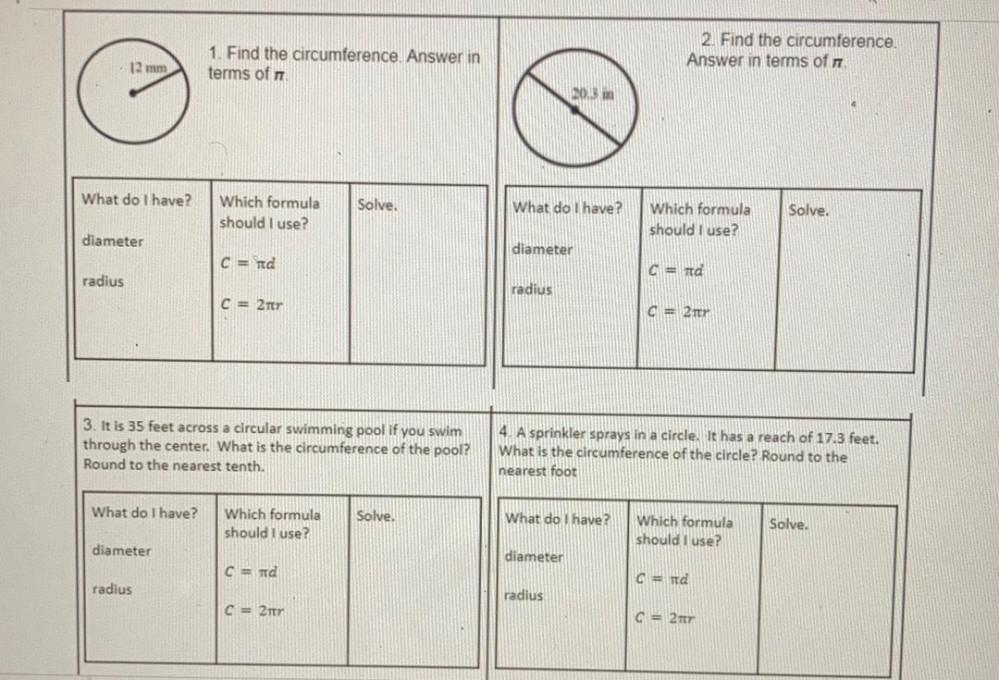Question:

# 2. Find the circumference Answer in terms of 1. Find the circumference. Answer in terms of 12 mm 20.3 What do I have? Which form2. Find the circumference Answer in terms of 1. Find the circumference. Answer in terms of 12 mm 20.3 What do I have? Which formula should I use? Solve. What do I have? Solve. Which formula should I use? diameter diameter C = id and radius radius C = 2tr 02tr 3. It is 35 feet across a circular swimming pool if you swim through the center. What is the circumference of the pool? Round to the nearest tenth. 4. A sprinkler sprays in a circle. It has a reach of 17.3 feet. What is the circumference of the circle? Round to the nearest foot What do I have? Which formula should I use? Solve. What do I have? Which formula should I use? Solve. diameter diameter C=id 0 = id radius radius C = 2 G = 2tr